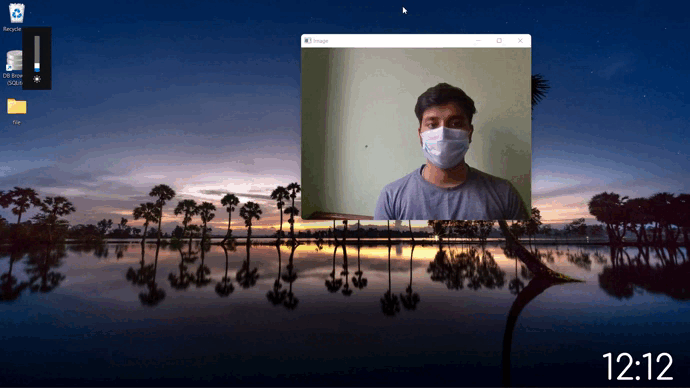GeeksforGeeks App
Open AppBrowser
Continue

# Brightness Control With Hand Detection using OpenCV in Python

In this article, we are going to make a Python project that uses OpenCV and Mediapipe to see hand gesture and accordingly set the brightness of the system from a range of 0-100.

We have used a HandTracking module that tracks all points on the hand and detects hand landmarks, calculate the distance between thumb tip and index fingertip and maps the distance between thumb tip and index fingertip with brightness range.

## Required Libraries

• Mediapipe: It is Google’s open-source framework, used for media processing. It is cross-platform or we can say it is platform friendly. It can run on Android, iOS, and the web that’s what Cross-platform means, to run everywhere.
`pip install mediapipe  `
• OpenCV: Itis a Python library that is designed to solve computer vision problems. OpenCV supports a wide variety of programming languages such as C++, Python, Java etc. Support for multiple platforms including Windows, Linux, and MacOS.
`pip install opencv-python`
• Screen-Brightness-Control: It is a python tool for controlling the brightness of your monitor. Supports Windows and most flavors of Linux.
`pip install screen-brightness-control`
• Numpy: It is a general-purpose array-processing package. It provides a high-performance multidimensional array object, and tools for working with these arrays. It is the fundamental package for scientific computing with Python.
`pip install numpy`

## Python3

 `# Importing Libraries``import` `cv2``import` `mediapipe as mp``from` `math ``import` `hypot``import` `screen_brightness_control as sbc``import` `numpy as np`

## Python3

 `# Initializing the Model``mpHands ``=` `mp.solutions.hands``hands ``=` `mpHands.Hands(``    ``static_image_mode``=``False``,``    ``model_complexity``=``1``,``    ``min_detection_confidence``=``0.75``,``    ``min_tracking_confidence``=``0.75``,``    ``max_num_hands``=``2``)`` ` `Draw ``=` `mp.solutions.drawing_utils`

Let us look into the parameters for the Hands Model:

Hands( static_image_mode=False, model_complexity=1 min_detection_confidence=0.75, min_tracking_confidence=0.75, max_num_hands=2 )

Where:

• static_image_mode: It is used to specify whether the input image must be static images or as a video stream. The default value is False.
• model_complexity: Complexity of the hand landmark model: 0 or 1. Landmark accuracy, as well as inference latency, generally go up with the model complexity. Default to 1.
• min_detection_confidence: It is used to specify the minimum confidence value with which the detection from the person-detection model needs to be considered as successful. Can specify a value in [0.0,1.0]. The default value is 0.5.
• min_tracking_confidence: It is used to specify the minimum confidence value with which the detection from the landmark-tracking model must be considered as successful. Can specify a value in [0.0,1.0]. The default value is 0.5.
• max_num_hands: Maximum number of hands to detect. Default it is 2.

### Step 3: Process the image and apply brightness based on the distance between thumb and index fingertip

Capture the frames continuously from the camera using OpenCV and Convert BGR image to an RGB image and make predictions using initialized hands model. Prediction made by the model is saved in the results variable from which we can access landmarks using results.multi_hand_landmarks and if hands are present in the frame, detect hand landmarks after that calculate the distance between thumb tip and index finger tip. Map the distance of thumb tip and index fingertip with brightness range i.e according to distance between them brightness of system will change.

## Python3

 `# Start capturing video from webcam``cap ``=` `cv2.VideoCapture(``0``)`` ` `while` `True``:``    ``# Read video frame by frame``    ``_,frame ``=` `cap.read()``     ` `    ``#Flip image ``    ``frame``=``cv2.flip(frame,``1``)``     ` `    ``# Convert BGR image to RGB image``    ``frameRGB ``=` `cv2.cvtColor(frame,cv2.COLOR_BGR2RGB)``     ` `    ``# Process the RGB image``    ``Process ``=` `hands.process(frameRGB)``     ` `    ``landmarkList ``=` `[]``    ``# if hands are present in image(frame)``    ``if` `Process.multi_hand_landmarks:``        ``# detect handmarks``        ``for` `handlm ``in` `Process.multi_hand_landmarks:``            ``for` `_id,landmarks ``in` `enumerate``(handlm.landmark):``                ``# store height and width of image``                ``height,width,color_channels ``=` `frame.shape``                 ` `                ``# calculate and append x, y coordinates``                ``# of handmarks from image(frame) to lmList``                ``x,y ``=` `int``(landmarks.x``*``width),``int``(landmarks.y``*``height)              ``                ``landmarkList.append([_id,x,y]) ``             ` `            ``# draw Landmarks``            ``Draw.draw_landmarks(frame,handlm,mpHands.HAND_CONNECTIONS)``     ` `    ``# If landmarks list is not empty``    ``if` `landmarkList !``=` `[]:``        ``# store x,y coordinates of (tip of) thumb  ``        ``x_1,y_1 ``=` `landmarkList[``4``][``1``],landmarkList[``4``][``2``]``         ` `        ``# store x,y coordinates of (tip of) index finger``        ``x_2,y_2 ``=` `landmarkList[``8``][``1``],landmarkList[``8``][``2``]``         ` `        ``# draw circle on thumb and index finger tip``        ``cv2.circle(frame,(x_1,y_1),``7``,(``0``,``255``,``0``),cv2.FILLED)``        ``cv2.circle(frame,(x_2,y_2),``7``,(``0``,``255``,``0``),cv2.FILLED)``         ` `        ``# draw line from tip of thumb to tip of index finger``        ``cv2.line(frame,(x_1,y_1),(x_2,y_2),(``0``,``255``,``0``),``3``)``         ` `        ``# calculate square root of the sum``        ``# of squares of the specified arguments.``        ``L ``=` `hypot(x_2``-``x_1,y_2``-``y_1)``         ` `        ``# 1-D linear interpolant to a function``        ``# with given discrete data points ``        ``# (Hand range 15 - 220, Brightness range 0 - 100),``        ``# evaluated at length.``        ``b_level ``=` `np.interp(L,[``15``,``220``],[``0``,``100``])``     ` `        ``# set brightness``        ``sbc.set_brightness(``int``(b_level))`` ` `    ``# Display Video and when 'q' is entered,``    ``# destroy the window``    ``cv2.imshow(``'Image'``, frame)``    ``if` `cv2.waitKey(``1``) & ``0xff` `=``=` `ord``(``'q'``):``        ``break`

## Python3

 `# Importing Libraries``import` `cv2``import` `mediapipe as mp``from` `math ``import` `hypot``import` `screen_brightness_control as sbc``import` `numpy as np`` ` `# Initializing the Model``mpHands ``=` `mp.solutions.hands``hands ``=` `mpHands.Hands(``    ``static_image_mode``=``False``,``    ``model_complexity``=``1``,``    ``min_detection_confidence``=``0.75``,``    ``min_tracking_confidence``=``0.75``,``    ``max_num_hands``=``2``)`` ` `Draw ``=` `mp.solutions.drawing_utils`` ` `# Start capturing video from webcam``cap ``=` `cv2.VideoCapture(``0``)`` ` `while` `True``:``    ``# Read video frame by frame``    ``_, frame ``=` `cap.read()`` ` `    ``# Flip image``    ``frame ``=` `cv2.flip(frame, ``1``)`` ` `    ``# Convert BGR image to RGB image``    ``frameRGB ``=` `cv2.cvtColor(frame, cv2.COLOR_BGR2RGB)`` ` `    ``# Process the RGB image``    ``Process ``=` `hands.process(frameRGB)`` ` `    ``landmarkList ``=` `[]``    ``# if hands are present in image(frame)``    ``if` `Process.multi_hand_landmarks:``        ``# detect handmarks``        ``for` `handlm ``in` `Process.multi_hand_landmarks:``            ``for` `_id, landmarks ``in` `enumerate``(handlm.landmark):``                ``# store height and width of image``                ``height, width, color_channels ``=` `frame.shape`` ` `                ``# calculate and append x, y coordinates``                ``# of handmarks from image(frame) to lmList``                ``x, y ``=` `int``(landmarks.x``*``width), ``int``(landmarks.y``*``height)``                ``landmarkList.append([_id, x, y])`` ` `            ``# draw Landmarks``            ``Draw.draw_landmarks(frame, handlm,``                                ``mpHands.HAND_CONNECTIONS)`` ` `    ``# If landmarks list is not empty``    ``if` `landmarkList !``=` `[]:``        ``# store x,y coordinates of (tip of) thumb``        ``x_1, y_1 ``=` `landmarkList[``4``][``1``], landmarkList[``4``][``2``]`` ` `        ``# store x,y coordinates of (tip of) index finger``        ``x_2, y_2 ``=` `landmarkList[``8``][``1``], landmarkList[``8``][``2``]`` ` `        ``# draw circle on thumb and index finger tip``        ``cv2.circle(frame, (x_1, y_1), ``7``, (``0``, ``255``, ``0``), cv2.FILLED)``        ``cv2.circle(frame, (x_2, y_2), ``7``, (``0``, ``255``, ``0``), cv2.FILLED)`` ` `        ``# draw line from tip of thumb to tip of index finger``        ``cv2.line(frame, (x_1, y_1), (x_2, y_2), (``0``, ``255``, ``0``), ``3``)`` ` `        ``# calculate square root of the sum of``        ``# squares of the specified arguments.``        ``L ``=` `hypot(x_2``-``x_1, y_2``-``y_1)`` ` `        ``# 1-D linear interpolant to a function``        ``# with given discrete data points``        ``# (Hand range 15 - 220, Brightness``        ``# range 0 - 100), evaluated at length.``        ``b_level ``=` `np.interp(L, [``15``, ``220``], [``0``, ``100``])`` ` `        ``# set brightness``        ``sbc.set_brightness(``int``(b_level))`` ` `    ``# Display Video and when 'q' is entered, destroy ``    ``# the window``    ``cv2.imshow(``'Image'``, frame)``    ``if` `cv2.waitKey(``1``) & ``0xff` `=``=` `ord``(``'q'``):``        ``break`

Output:My Personal Notes arrow_drop_up